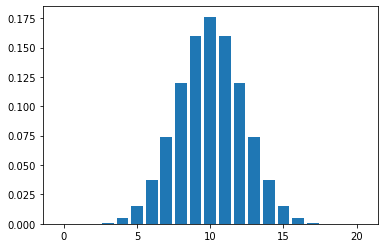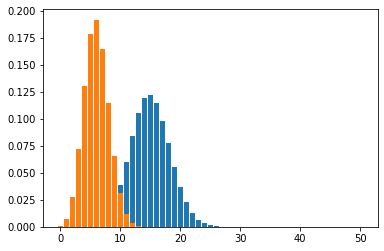Learning library

For all the self-taught geeks out there, here is our content library with most of the learning materials we have produced throughout the years.

It makes sense to start learning by reading and watching videos about fundamentals and how things work.

Data Science and Machine Learning - 16 wks

Full-Stack Software Developer - 16w

Search from all Lessons

Social & live learning

The most efficient way to learn: Join a cohort with classmates just like you, live streams, impromptu coding sessions, live tutorials with real experts, and stay motivated.

← Back to Lessons
Edit on Github
Open in Collab

# Mean and Std with Python

### Mean and Standard Deviation¶

$\text{mean} = \mu = \frac{1}{n}\sum_{i = 1}^n x_i$
$\text{standard deviation} = \sigma = \sqrt{\frac{1}{n}\sum_{i = 1}^n (x_i - \mu)^2}$
In [ ]:
#mean of a list
x = [3, 5, 7, 9, 11]
np.mean(x)

Out[ ]:
7.0
In [ ]:
#standard deviation of list
np.std(x)

Out[ ]:
2.8284271247461903
In [ ]:
#Binomial distribution with n = 20, p = 0.5
b = stats.binom(20, 0.5)

In [ ]:
#mean
b.mean()

Out[ ]:
10.0
In [ ]:
#plot
plt.bar(range(21), b.pmf(range(21)))

Out[ ]:
<BarContainer object of 21 artists>In [ ]:
#another example
#Binomial n = 50, p = 0.3
ex2 = stats.binom(50, 0.3)
ex3 = stats.binom(20, 0.3)

In [ ]:
#mean
ex2.mean()

Out[ ]:
15.0
In [ ]:
ex3.mean()

Out[ ]:
6.0
In [ ]:
ex3.std()

Out[ ]:
2.0493901531919194
In [ ]:
#deviation
ex2.std()

Out[ ]:
3.24037034920393
In [ ]:
#plot
plt.bar(range(51), ex2.pmf(range(51)))
plt.bar(range(21), ex3.pmf(range(21)))

Out[ ]:
<BarContainer object of 21 artists>